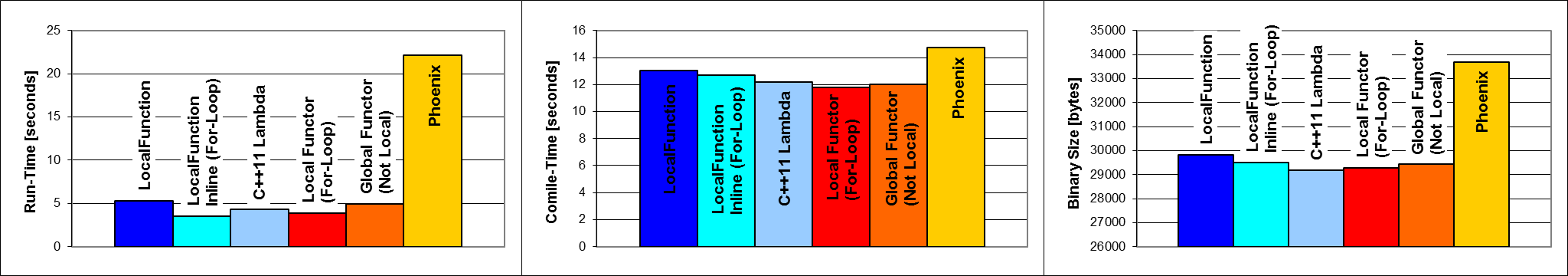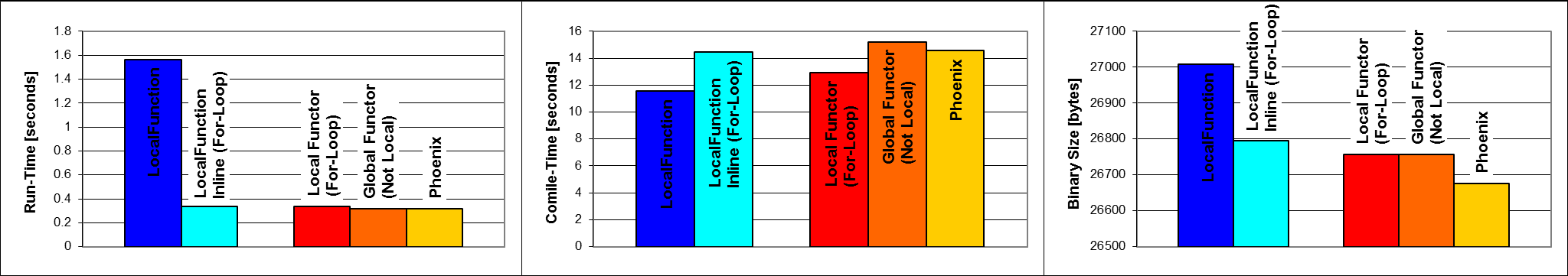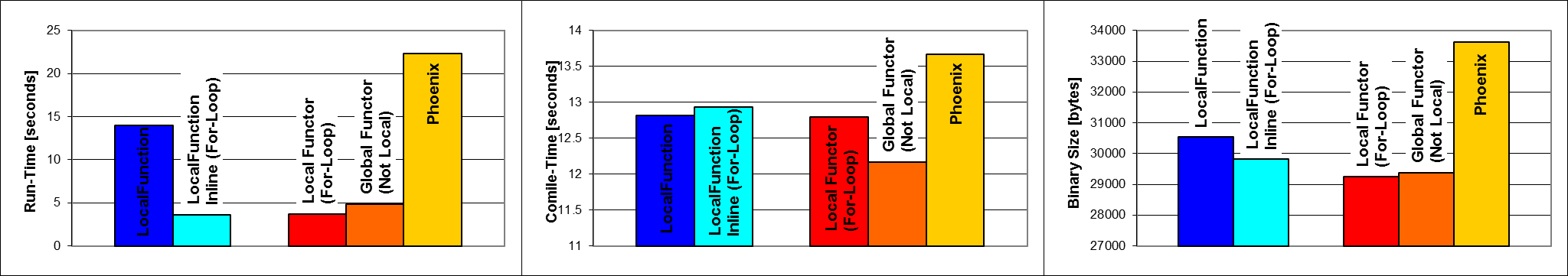#Boost C++ Libraries

...one of the most highly regarded and expertly designed C++ library projects in the world.

This is the documentation for a snapshot of the develop branch, built from commit 4e6c143b17.

## Annex: Alternatives

This section compares the features offered by this library with similar features offered by C++ and by other libraries.

#### Features

The following table compares local function features.

Local Function Feature

Boost.LocalFunction

C++11 Lambda Function (Not C++03)

Local Functor

Global Functor (Not Local)

Boost.Phoenix

Can be defined locally

Yes.

Yes.

Yes.

No. Therefore this not really an alternative implementation of local functions but it is listed here just for comparison.

Yes.

Can be defined using C++ statement syntax

Yes. Plus eventual compiler errors and debugging retain their usual meaning and format.

Yes. Plus eventual compiler errors and debugging retain their usual meaning and format.

Yes. Plus eventual compiler errors and debugging retain their usual meaning and format.

Yes. Plus eventual compiler errors and debugging retain their usual meaning and format.

No (it uses C++ expression template syntax).

Can be defined within expressions

No. It can be defined only within declarations.

Yes (plus the local function can be unnamed).

No. It can be defined only within declarations.

No. It can be defined only within declarations.

Yes (plus the local function can be unnamed).

Can be passed as template parameter (e.g., to STL algorithms)

Yes. The C++03 standard does not allow to pass local types as template parameters (see [N2657]) but this library implements a "trick" to get around this limitation (see the Implementation section).

Yes.

No on C++03 compilers (but yes on C++11 compilers and some compilers like MSVC 8.0, see [N2657]).

Yes.

Yes.

Access variables in scope

Yes. The variable names are repeated in the function declaration so they can be bound by value, by constant value, by reference, and by constant reference (the object `this` can also be bound using `this_`).

Yes. The variable names are repeated in the function declaration (plus there is a short-hand syntax to bind all variables in scope at once) so they can be bound by constant value and by reference (the object `this` can also be bound). However, variables cannot be bound by constant references (see below).

No. Programmers must manually program functor data members and explicitly specify their types to access variables in scope.

No. Programmers must manually program functor data members and explicitly specify their types to access variables in scope.

Yes. Variables in scope are accessible as usual within expressions (plus `boost::phoenix::let` can be used to bind variables by constant reference).

Polymorphic in the function parameter type

No (local functions cannot be function templates).

No (C++11 lambdas cannot be function templates).

No (local classes cannot have member function templates).

Yes.

Yes.

C++11 Lambda Function

C++11 lambda functions have most of the features of this library plus some additional feature (see also the example in the Introduction section):

• C++11 lambda functions can be defined within expressions while this library local functions can only be defined at declaration scope.
• C++11 lambda functions are only supported by the C++11 standard so they are not supported by all C++ compilers. This library local functions can be programmed also on C++03 compilers (and they have performances comparable to C++11 lambda functions on C++11 compilers).
• C++11 lambda functions do not allow to bind variables in scope by constant reference. Because a variable cannot be bound by constant reference, C++11 lambda functions can bind a variable by constant only if the variable is `CopyConstructible` and the binding requires a (potentially expensive) extra copy operation. Constant reference binding is instead supported by this library.
• C++11 lambda functions do not allow to bind data members selectively without binding also the object `this` while this library local functions can bind either selected data members or the entire object `this` (using `this_`).
• C++11 lambda functions provide a short-hand syntax to bind all variables in scope at once (`&` or `=`) while this library local function always require to bind variables naming them one-by-one.

For example, for non-copyable objects (see also `noncopyable_cxx11_lambda_error.cpp` and `noncopyable_local_function.cpp`):

C++11 Lambda Function

Boost.LocalFunction

```struct n: boost::noncopyable {
int i;
n(int _i): i(_i) {}
};

int main(void) {
n x(-1);

auto f = [x](void) {    // Error: x is non-copyable, but if
assert(x.i == -1);  // bind `&x` then `x` is not constant.
};
f();

return 0;
}
```

```struct n: boost::noncopyable {
int i;
n(int _i): i(_i) {}
};
BOOST_TYPEOF_REGISTER_TYPE(n) // Register for `bind& x` below.

int main(void) {
n x(-1);

void BOOST_LOCAL_FUNCTION(const bind& x) {  // OK: No copy
assert(x.i == -1);                      // and constant.
} BOOST_LOCAL_FUNCTION_NAME(f)
f();

return 0;
}
```

Or, for objects with expensive copy operations (see also `expensive_copy_cxx11_lambda.cpp` and `expensive_copy_local_function.cpp`):

C++11 Lambda Function

Boost.LocalFunction

```struct n {
int i;
n(int _i): i(_i) {}
n(n const& x): i(x.i) { // Some time consuming copy operation.
for (unsigned i = 0; i < 10000; ++i) std::cout << '.';
}
};

int main(void) {
n x(-1);

auto f = [x]() {        // Problem: Expensive copy, but if bind
assert(x.i == -1);  // by `&x` then `x` is not constant.
};
f();

return 0;
}
```

```struct n {
int i;
n(int _i): i(_i) {}
n(n const& x): i(x.i) { // Some time consuming copy operation.
for (unsigned i = 0; i < 10000; ++i) std::cout << '.';
}
};
BOOST_TYPEOF_REGISTER_TYPE(n) // Register for `bind& x` below.

int main(void) {
n x(-1);

void BOOST_LOCAL_FUNCTION(const bind& x) {  // OK: No copy expensive
assert(x.i == -1);                      // copy but constant.
} BOOST_LOCAL_FUNCTION_NAME(f)
f();

return 0;
}
```

When constant binding functionality is needed for C++11 lambda functions, the best alternative might be to bind an extra local variable declared constant and initialized to the original variable (for example, see constant blocks implemented with C++11 lambda functions in the Examples section).

Local Functor

The following example compares local functions with C++ local functors (see also `add_local_functor.cpp` and `add.cpp`):

Local Functor

Boost.LocalFunction

```int main(void) {
int sum = 0, factor = 10;

struct local_add { // Unfortunately, boilerplate code to program the class.
local_add(int& _sum, int _factor): sum(_sum), factor(_factor) {}

inline void operator()(int num) { // Body uses C++ statement syntax.
sum += factor * num;
}

private: // Unfortunately, cannot bind so repeat variable types.
int& sum; // Access `sum` by reference.
const int factor; // Make `factor` constant.

int nums[] = {2, 3};
// Unfortunately, cannot pass as template parameter to `std::for_each`.
for(size_t i = 0; i < 2; ++i) add(nums[i]);

BOOST_TEST(sum == 60);
return boost::report_errors();
}
```

```int main(void) {                            // Some local scope.
int sum = 0, factor = 10;               // Variables in scope to bind.

void BOOST_LOCAL_FUNCTION(const bind factor, bind& sum, int num) {
sum += factor * num;

add(1);                                 // Call the local function.
int nums[] = {2, 3};
std::for_each(nums, nums + 2, add);     // Pass it to an algorithm.

BOOST_TEST(sum == 60);                  // Assert final summation value.
return boost::report_errors();
}
```

Global Functor

The following example compares local functions with C++ global functors (see also `add_global_functor.cpp` and `add.cpp`):

Global Functor

Boost.LocalFunction

```// Unfortunately, cannot be defined locally (so not a real alternative).
struct global_add { // Unfortunately, boilerplate code to program the class.
global_add(int& _sum, int _factor): sum(_sum), factor(_factor) {}

inline void operator()(int num) { // Body uses C++ statement syntax.
sum += factor * num;
}

private: // Unfortunately, cannot bind so repeat variable types.
int& sum; // Access `sum` by reference.
const int factor; // Make `factor` constant.
};

int main(void) {
int sum = 0, factor = 10;

int nums[] = {2, 3};
std::for_each(nums, nums + 2, add); // Passed as template parameter.

BOOST_TEST(sum == 60);
return boost::report_errors();
}
```

```int main(void) {                            // Some local scope.
int sum = 0, factor = 10;               // Variables in scope to bind.

void BOOST_LOCAL_FUNCTION(const bind factor, bind& sum, int num) {
sum += factor * num;

add(1);                                 // Call the local function.
int nums[] = {2, 3};
std::for_each(nums, nums + 2, add);     // Pass it to an algorithm.

BOOST_TEST(sum == 60);                  // Assert final summation value.
return boost::report_errors();
}
```

However, note that global functors do not allow to define the function locally so they are not a real alternative implementation of local functions.

Boost.Phoenix

The following example compares local functions with Boost.Phoenix (see also `add_phoenix.cpp` and `add.cpp`):

Boost.Phoenix

Boost.LocalFunction

```int main(void) {
using boost::phoenix::let;
using boost::phoenix::local_names::_f;
using boost::phoenix::cref;
using boost::phoenix::ref;
using boost::phoenix::arg_names::_1;

int sum = 0, factor = 10;
int nums[] = {1, 2, 3};

// Passed to template, `factor` by constant, and defined in expression.
std::for_each(nums, nums + 3, let(_f = cref(factor))[
// Unfortunately, body cannot use C++ statement syntax.
ref(sum) += _f * _1, _1 // Access `sum` by reference.
]);

BOOST_TEST(sum == 60);
return boost::report_errors();
}
```

```int main(void) {                            // Some local scope.
int sum = 0, factor = 10;               // Variables in scope to bind.

void BOOST_LOCAL_FUNCTION(const bind factor, bind& sum, int num) {
sum += factor * num;

add(1);                                 // Call the local function.
int nums[] = {2, 3};
std::for_each(nums, nums + 2, add);     // Pass it to an algorithm.

BOOST_TEST(sum == 60);                  // Assert final summation value.
return boost::report_errors();
}
```

The comparison in this section does not include the Boost.Lambda library because that library is obsolete and it was replaced by Boost.Phoenix. The Boost.Phoenix library version 3.0 is used for this comparison.

#### Performances

The following tables compare run-times, compile-times, and binary sizes for the different alternatives to local functions presented in this section.

Overall, this library has compile-times and generates binary sizes similar to the ones of the other approaches. This library run-times on C++03 compilers were measured to be larger than other approaches when compiler optimization is enabled (using ```bjam release ...```). However, on compilers that allow to pass local types as template parameters (e.g., MSVC 8.0 or GCC 4.5.3 with C++11 features enabled `-std=c++0x`, see also [N2657] and Boost.Config's `BOOST_NO_LOCAL_CLASS_TEMPLATE_PARAMETERS`) this library automatically generates optimized code that runs as fast as the fastest of the other approaches (see the "Boost.LocalFunction" approach below). When this library local function is specified `inline` (see the "Boost.LocalFunction Inline" approach below and the Advanced Topics section) its run-times are always comparable to both the "Local Functor" and "Global Functor" approaches. However, in these cases the local function cannot be portably passed as template parameter (see [N2657] and Boost.Config's `BOOST_NO_LOCAL_CLASS_TEMPLATE_PARAMETERS`) so `std::for_each` is replaced by a for-loop (on MSVC the for-loop, and not the local function in fact the same applies to local functors, was measured to have worst performances than using `std::for_each`). Finally, this library run-times are always among the fastest when no compiler optimization is enabled (using ```bjam debug ...```).

NoteThe run-time performances of this library local functions are explained because on C++03 compliant compilers (e.g., GCC 4.5.3 without `-std=c++0x`) this library needs to use a function pointer in order to portably pass the local function class as a template parameter (see [N2657] and the Implementation section). For all tested compilers, this function pointer prevents the compiler optimization algorithms from inlining the local function calls. Instead, the functors used by other approaches (e.g., Boost.Phoenix) have been observed to allow all tested compilers to inline all the function calls for optimization. This run-time performance cost is not present on compilers that allow to pass local types as template parameters (e.g., MSVC 8.0 or GCC 4.5.3 with C++11 features enabled `-std=c++0x`, see Boost.Config's `BOOST_NO_LOCAL_CLASS_TEMPLATE_PARAMETERS`) because this library does not have to use the extra function pointer to implement the local function call (it directly passes the local class type as template parameter).

This run-time performance cost on C++03 compilers might or might not be an issue depending on the performance requirements of specific applications. For example, an application might already be using a number of indirect function calls (function pointers, virtual functions, etc) for which the overhead added by using the one extra function pointer required by the local function call might not be noticeable within the overall program run-time.

Finally, note that only a very simple local function body with just a single instruction was used for the anaylsis presented here (see the source files below). The authors have not studied how this library and the other approaches will perform with respect to each other when a more complex set of instructions is programmed for the local function body (e.g., if a more complex set of instructions in the local function body were to inhibit some compiler from inlining function objects also other approaches like C++11 lambda functions and Boost.Phoenix could start to show higher run-times even when optimization is enabled).

The following commands were executed from the library example directory to measure compile-time, binary size, and run-time respectively:

```> touch <FILE_NAME>.cpp                             # force recompilation
> python chrono.py bjam {release|debug} <FILE_NAME> # compile-time
> size <FILE_NAME>                                  # binary size
> ./<FILE_NAME>                                     # run-time
```

The local function was called `1e8` times to add together all the elements of a vector and the run-time was measured using Boost.Chrono averaging over `10` executions of the vector summation (see the source files below).

Legend

Approach

Source FileBoost.LocalFunction inlineC++11 Lambda Function [a]Local FunctorGlobal Functor[a] Measurements available only for C++11 compilers.

GCC 4.5.3 With C++11 Lambda Functions and "Local Classes as Template Parameters" (`bjam cxxflags=-std=c++0x ...`)

Compiled with `bjam release ...` for maximum optimization (`-O3 -finline-functions`)Compiled with `bjam debug ...` for no optimization (`-O0 -fno-inline`)MSVC 8.0 With "Local Classes as Template Parameters" (Without C++11 Lambda Functions)

Compiled with `bjam release ...` for maximum optimization (`/O2 /Ob2`)Compiled with `bjam debug ...` for no optimization (`/Od /Ob0`)GCC 4.3.4 With C++03 Only (Without C++11 Lambda Functions and Without "Local Classes as Template Parameters")

Compiled with `bjam release ...` for maximum optimization (`-O3 -finline-functions`)Compiled with `bjam debug ...` for no optimization (`-O0 -fno-inline`)